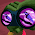## вторник, 24 декабря 2013 г.

### Codility. Train. Frog-Jmp ★

A small frog wants to get to the other side of the road. The frog is currently located at position X and wants to get to a position greater than or equal to Y. The small frog always jumps a fixed distance, D.
Count the minimal number of jumps that the small frog must perform to reach its target.
Write a function:
def solution(X, Y, D)
that, given three integers X, Y and D, returns the minimal number of jumps from position X to a position equal to or greater than Y.
For example, given:
```  X = 10
Y = 85
D = 30```
the function should return 3, because the frog will be positioned as follows:
• after the first jump, at position 10 + 30 = 40
• after the second jump, at position 10 + 30 + 30 = 70
• after the third jump, at position 10 + 30 + 30 + 30 = 100
Assume that:
• X, Y and D are integers within the range [1..1,000,000,000];
• X ≤ Y.
Complexity:
• expected worst-case time complexity is O(1);
• expected worst-case space complexity is O(1).

Solution:
```import math
def solution(X, Y, D):
return int(math.ceil((Y-X)/float(D)))
```

#### 2 комментария:

1.What language did you use? Java?

2.